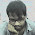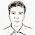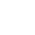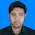### Fibonacci : Sum of even-valued terms of Fibonacci Sequence

Each new term in the Fibonacci sequence is generated by adding the previous two terms. By starting with 1 and 2, the first 10 terms will be:
1, 2, 3, 5, 8, 13, 21, 34, 55, 89...
By considering the terms in the Fibonacci sequence whose values do not exceed four million, find the sum of the even-valued terms.

Source Code in C:

#include<stdio.h>
void main()
{
long f1 = 1, f2 = 2, f3, range,sum=0;
clrscr();
printf("Enter the maximum range: ");
scanf("%ld", &range);
while(1)
{
f3 = f1 + f2;
if(f3 > range)
break;
if (f3%2==0)
sum+=f3;
if (f3==3) sum+=2;
f1 = f2;
f2 = f3;
}
printf("The sum of all even digit : %ld",sum);
getch();
}

1.2.3./*****************************************
Converted By Forhad Parvez
CSE-55
ID: 201720297
******************************************/

#include

using namespace std;

class fibo{
public:
int fbcalc(int range){
long f1 = 1, f2 = 2, f3, sum=0;
while(1) {
f3 = f1 + f2;
if(f3 > range)
break;
if (f3%2==0)
sum+=f3;
if (f3==3) sum+=2;
f1 = f2;
f2 = f3;
}

return sum;
}

};

int main()
{
int a, val;
fibo obj;
cout<<"Enter A range for fibonacci calculation : ";
cin>>a;
val=obj.fbcalc(a);
cout<<"The summation of the range is : "<<val;
return 0;
}

4.5.test only

6.#include
using namespace std;

class homework
{
public:
int result = 0;

int method (int range)
{
int n1 = 0, n2 = 1, n3 = 1;
while (n3 <= range)
{
n3 = n1 + n2;
if (n3 % 2 == 0)
{
result = result + n3;
}
n1 = n2;
n2 = n3;
}
return result;
}

void perameter (int range)
{
cout << "The result is = " << method (range);
}

void perameterless ()
{
int range;
cout << "Please input number as RANGE = ";
cin >> range;
cout << "The result is = " << method (range);
}

int returnable (int range)
{
return method (range);
}
};

int main ()
{
homework hw;
int range;
char method;

cout <<"To sum all of the even number from this series (1,2,3,5,8,13,21......RANGE) chouse any option\n";
cout << "(a) Using method\n";
cout << "(b) Method with perameter\n";
cout << "(c) Method without perameter\n";
cout << "(d) Method without returntype and parameter\n";

select:
cout << "\n Type a/b/c/d = ";
cin >> method;
cout << "\n";

switch (method)
{
case 'a':
cout << "You select METHOD option \n";
cout << "Please input number as RANGE = ";
cin >> range;
cout << "The result is = " << hw.method (range);
break;

case 'b':
cout << "You select METHOD WITH PARAMETER option \n";
cout << "Please input number as RANGE = ";
cin >> range;
hw.perameter (range);
break;

case 'c':
cout << "You select METHOD WITHOUT PARAMETER option \n";
hw.perameterless ();
break;

case 'd':
cout << "You select METHOD WITH RETURNTYPE & PARAMETER option \n";
cout << "Please input number as RANGE = ";
cin >> range;
cout << "The result is = " << hw.returnable (range);
break;

default:
cout << "Your input not valid. it should be a/b/c/d\n";
goto select;
break;
}
}

7.8.Name: Sayeka Jannat
ID: 201720097
CSE-55

#include
using namespace std;
class fibo
{
public:
int x=1, y=2, z,sum=0;
int add (int range){

while(1){
z=x+y;
if(z>range)
break;
if (z%2==0)
sum +=z;
if (z==3)sum+=2;
x=y;
y=z;
}
return sum;
}
};

int main() {
int n;
fibo c;
cout<<"Enput a number : ";
cin>>n;
cout<<"The summation of every even number is : "<<c.add(n);
return 0;
}

9.Name: Sayeka Jannat
ID: 201720097
CSE-55

#include
using namespace std;
class fibo
{
public:
int x=1, y=2, z,sum=0;
int add (int range){

while(1){
z=x+y;
if(z>range)
break;
if (z%2==0)
sum +=z;
if (z==3)sum+=2;
x=y;
y=z;
}
return sum;
}
};

int main() {
int n;
fibo c;
cout<<"Enput a number : ";
cin>>n;
cout<<"The summation of every even number is : "<<c.add(n);
return 0;
}

10.#include
using namespace std;

class homework
{
public:
int result = 0;

int method (int range)
{
int n1 = 0, n2 = 1, n3 = 1;
while (n3 <= range)
{
n3 = n1 + n2;
if (n3 % 2 == 0)
{
result = result + n3;
}
n1 = n2;
n2 = n3;
}
return result;
}

void perameter (int range)
{
cout << "The result is = " << method (range);
}

void perameterless ()
{
int range;
cout << "Please input number as RANGE = ";
cin >> range;
cout << "The result is = " << method (range);
}

int returnable (int range)
{
return method (range);
}
};

int main ()
{
homework hw;
char method;
int range;

cout <<"To sum all of the even number from this series (1,2,3,5,8,13,21......RANGE) choce any option\n";
cout << "(a) Using method\n";
cout << "(b) Method with parameter\n";
cout << "(c) Method without parameter\n";
cout << "(d) Method without return type and parameter\n";

select:
cout << "\n Type a/b/c/d = ";
cin >> method;
cout << "\n";

switch (method)
{
case 'a':
cout << "You select METHOD option \n";
cout << "Please input number as RANGE = ";
cin >> range;
cout << "The result is = " << hw.method (range);
break;

case 'b':
cout << "You select METHOD WITH PARAMETER option \n";
cout << "Please input number as RANGE = ";
cin >> range;
hw.perameter (range);
break;

case 'c':
cout << "You select METHOD WITHOUT PARAMETER option \n";
hw.perameterless ();
break;

case 'd':
cout << "You select METHOD WITH RETURNTYPE & PARAMETER option \n";
cout << "Please input number as RANGE = ";
cin >> range;
cout << "The result is = " << hw.returnable (range);
break;

default:
cout << "Your input not valid. it should be a/b/c/d\n";
goto select;
break;
}
}

11.Converted By Md. Al-Hasan
CSE-56th
ID:- 201730011
**************************

#include

using namespace std;

class calc
{
public:
int sum(int x,int y)
{
return x+y;
}

int sub(int x,int y)

{
return x-y;
}
};

int main()
{
calc c;
int x,y;
cin>>x>>y;

cout<<c.sum(x,y)<<"\n";
cout<<c.sub(x,y)<<"\n";
}

12.//Taslima Akter
//ID-201720079
//Batch-55

#include
using namespace std;
class fibo
{
public:
int f1=1,f2=2,f3,sum=0;
{
while(1)
{
f3=f1+f2;
if(f3>range)
break;
if(f3%2==0)
sum+=f3;
if(f3==3)sum+=2;
f1=f2;
f2=f3;

}
return sum;

}
};
int main()
{
int n;
fibo c;
cout<<"enter number:";
cin>>n;
cout<<"the sum of all even digit"<<c.add(n);
return 0;
}

13.Name: Md. Johorul Islam Jewel
ID: 201720237
Batch : Diploma - 55

14.Rezvi Akter
Cse-56th
ID-201730081
Sec-B
*************

#include
using namespace std;

class homework
{
public:
int result = 0;

int method (int range)
{
int n1 = 0, n2 = 1, n3 = 1;
while (n3 <= range)
{
n3 = n1 + n2;
if (n3 % 2 == 0)
{
result = result + n3;
}
n1 = n2;
n2 = n3;
}
return result;
}

void perameter (int range)
{
cout << "The result is = " << method (range);
}

void perameterless ()
{
int range;
cout << "Please input number as RANGE = ";
cin >> range;
cout << "The result is = " << method (range);
}

int returnable (int range)
{
return method (range);
}
};

int main ()
{
homework hw;
int range;
char method;

cout <<"To sum all of the even number from this series (1,2,3,5,8,13,21......RANGE) chouse any option\n";
cout << "(a) Using method\n";
cout << "(b) Method with perameter\n";
cout << "(c) Method without perameter\n";
cout << "(d) Method without returntype and parameter\n";

select:
cout << "\n Type a/b/c/d = ";
cin >> method;
cout << "\n";

switch (method)
{
case 'a':
cout << "You select METHOD option \n";
cout << "Please input number as RANGE = ";
cin >> range;
cout << "The result is = " << hw.method (range);
break;

case 'b':
cout << "You select METHOD WITH PARAMETER option \n";
cout << "Please input number as RANGE = ";
cin >> range;
hw.perameter (range);
break;

case 'c':
cout << "You select METHOD WITHOUT PARAMETER option \n";
hw.perameterless ();
break;

case 'd':
cout << "You select METHOD WITH RETURNTYPE & PARAMETER option \n";
cout << "Please input number as RANGE = ";
cin >> range;
cout << "The result is = " << hw.returnable (range);
break;

default:
cout << "Your input not valid. it should be a/b/c/d\n";
goto select;
break;
}
}

15.Rezvi Akter
Cse-56th
ID-201730081
Sec-B
************

#include
using namespace std;

class homework
{
public:
int result = 0;

int method (int range)
{
int n1 = 0, n2 = 1, n3 = 1;
while (n3 <= range)
{
n3 = n1 + n2;
if (n3 % 2 == 0)
{
result = result + n3;
}
n1 = n2;
n2 = n3;
}
return result;
}

void perameter (int range)
{
cout << "The result is = " << method (range);
}

void perameterless ()
{
int range;
cout << "Please input number as RANGE = ";
cin >> range;
cout << "The result is = " << method (range);
}

int returnable (int range)
{
return method (range);
}
};

int main ()
{
homework hw;
int range;
char method;

cout <<"To sum all of the even number from this series (1,2,3,5,8,13,21......RANGE) chouse any option\n";
cout << "(a) Using method\n";
cout << "(b) Method with perameter\n";
cout << "(c) Method without perameter\n";
cout << "(d) Method without returntype and parameter\n";

select:
cout << "\n Type a/b/c/d = ";
cin >> method;
cout << "\n";

switch (method)
{
case 'a':
cout << "You select METHOD option \n";
cout << "Please input number as RANGE = ";
cin >> range;
cout << "The result is = " << hw.method (range);
break;

case 'b':
cout << "You select METHOD WITH PARAMETER option \n";
cout << "Please input number as RANGE = ";
cin >> range;
hw.perameter (range);
break;

case 'c':
cout << "You select METHOD WITHOUT PARAMETER option \n";
hw.perameterless ();
break;

case 'd':
cout << "You select METHOD WITH RETURNTYPE & PARAMETER option \n";
cout << "Please input number as RANGE = ";
cin >> range;
cout << "The result is = " << hw.returnable (range);
break;

default:
cout << "Your input not valid. it should be a/b/c/d\n";
goto select;
break;
}
}

16.I am jakir ,ID-201730574,Program not done !!!
#include
using namespace std;
class fibonac{
public:
int a,b,c,sum;
void generate(int);
};
void fibonac::generate(int n){
a=1; b=2; sum=0;
while(1){
c=a+b;
if(c>n)
break;
if(c%2==0)
sum+=c;
if(c==3)
sum+=2;
a=b;
b=c;
}

}

int main()
{
int d, val;
fibo ob;
cout<<"Enter A range for fibonacci calculation : ";
cin>>d;
val= ob.generate(d);
cout<<"The summation of the range is : "<<val;
return 0;
}